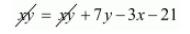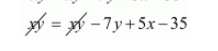Deepak Scored 45->99%ile with Bounce Back Crack Course. You can do it too!

# The area of a rectangle remains the same if the length is increased by 7 meters and the breadth is decreased by 3 meters.

Question:

The area of a rectangle remains the same if the length is increased by 7 meters and the breadth is decreased by 3 meters. The area remains unaffected if the length is decreased by 7 meters and breadth in increased by 5 meters. Find the dimensions of the rectangle.

Solution:

Let the length and breadth of the rectangle be $x$ and $y$ units respectively

Then, area of rectangle $=x y$ square units

If length is increased by 7 meters and breadth is decreased by 3 meters when the area of a rectangle remains the same

Therefore,

$x y=(x+7)(y-3)$

$x y=x y+7 y-3 x-21$$3 x-7 y+21=0 \cdots(i)$

If the length is decreased by 7 meters and breadth is increased by 5 meters when the area remains unaffected, then

$x y=(x-7)(y+5)$

$x y=x y-7 y+5 x-35$$0=5 x-7 y-35 \cdots(i i)$

Thus we get the following system of linear equation

$3 x-7 y+21=0$

$5 x-7 y-35=0$

By using cross-multiplication, we have

$\frac{x}{(-7 \times-35)-(-7 \times 21)}=\frac{-y}{(3 \times-35)-(5 \times 21)}=\frac{1}{(3 \times-7)-(5 \times-7)}$

$\frac{x}{245+147}=\frac{-y}{-105-105}=\frac{1}{-21+35}$

$\frac{x}{392}=\frac{-y}{-210}=\frac{1}{14}$

$x=\frac{392}{14}$

$x=28$

and

$y=\frac{210}{14}$

$y=15$

Hence, the length of rectangle is 28 meters

The breadth of rectangle is 15 meters